Open in App
Not now

# How to Read Data From Formula Cell in Excel Sheet using Selenium and Apache POI?

• Difficulty Level : Hard
• Last Updated : 12 May, 2022

The Apache POI is one of the most popular java libraries for selenium data-driven testing. It is mainly used for handling all types of Microsoft documents such as MS Word, and MS Excel. MS word and others. Apache POI is an open-source library and POI stands for “Poor Obfuscation Implementation”.

### Getting Started with Apache POI

We need to download the Apache POI to get started with the Apache POI library.

• Create the Maven project and install the Selenium driver

Geeks, for creating a maven project with selenium check this article How to Create a Selenium Maven Project with Eclipse to Open Chrome Browser?

• Add the Apache POI dependency mvn repository
• Go to https://mvnrepository.com/ and search for Apache POI and select the latest stable version, also install the poi-OOXML dependency.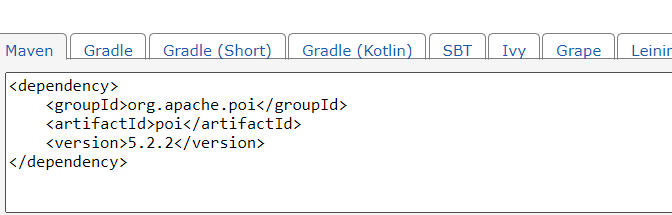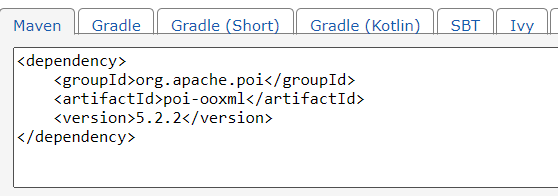• Copy the dependency and paste it into the pom.xml file in the maven project.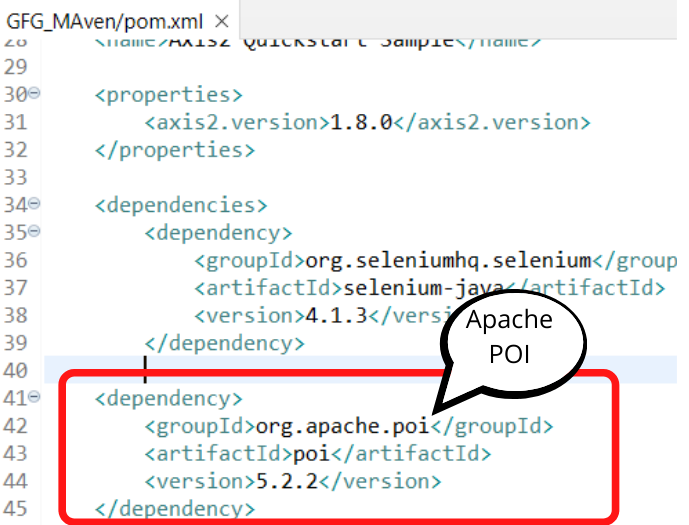### Creating Excel Sheet for Testing

Now create the Excel sheet with two columns Salary and bonus and calculate the Total using the Formula. If we try to read the data from the Formula table we usually occur errors. So we try to read the data in the formula data type itself.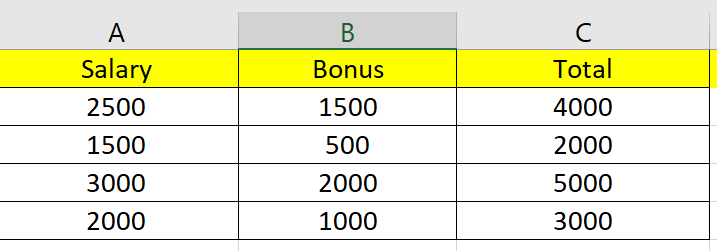Save the Excel File and paste the location in the file input stream in the code.

## Java

 `package` `GFG_Maven.GFG_MAven;`` ` `import` `org.testng.annotations.Test;``import` `java.io.FileInputStream;``import` `java.io.IOException;`` ` `import` `java.util.Iterator;`` ` `import` `org.apache.poi.ss.usermodel.Cell;``import` `org.apache.poi.ss.usermodel.Row;`` ` `import` `org.apache.poi.xssf.usermodel.XSSFSheet;``import` `org.apache.poi.xssf.usermodel.XSSFWorkbook;`` ` `public` `class` `Geeks {``    ``@Test``    ``public` `void` `geekforgeeks() ``throws` `IOException{``             ` `          ``FileInputStream file = ``new` `FileInputStream(``"C:\\Users\\ADMIN\\Desktop\\geeks.xlsx"``);``            ``XSSFWorkbook wb=``new` `XSSFWorkbook(file);``            ``XSSFSheet sheet=wb.getSheet(``"Sheet1"``);``      ` `            ``// Iterate each row one by one``            ``Iterator rIterator = sheet.iterator();``            ``while` `(rIterator.hasNext()) ``            ``{``                ``Row row = rIterator.next();``                 ` `                  ``// For each row, iterate through all the columns``                ``Iterator Cell = row.cellIterator();``                  ` `                ``while` `(Cell.hasNext()) ``                ``{``                    ``Cell cell = Cell.next();``                     ` `                      ``// Check the cell type``                    ``switch``(cell.getCellType())``                    ``{``                    ``case` `STRING:``                        ``System.out.print(cell.getStringCellValue());``                        ``break``;``                         ` `                    ``case` `NUMERIC:``                        ``System.out.print(cell.getNumericCellValue()); ``                        ``break``;``                         ` `                    ``case` `FORMULA:``                        ``System.out.print(cell.getNumericCellValue());``                        ``break``;``                    ``}``                    ``System.out.print(``"|"``);``                ``}``                ``System.out.println();              ``            ``}``            ``wb.close();``            ``file.close();``    ``}``}`

### Output

After Executing the program, we will get the all data from Excel.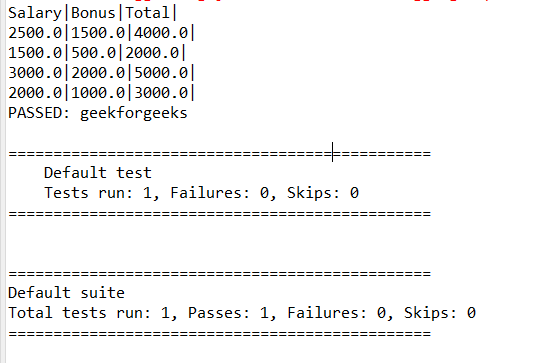### Code Explanation

• Creating the file input stream paste the location of the Excel file.

FileInputStream file = new FileInputStream(“file location”);

• Now open the file in the XSSFworkbook and open get the sheet1.
• Get the number of rows and columns.
• Iterate and get each cell value.
• Check the type of data in the switch case.
• Here we are given the FORMULA type for reading the cell value in the Formula column.

case FORMULA:
System.out.print(cell.getNumericCellValue());

My Personal Notes arrow_drop_up